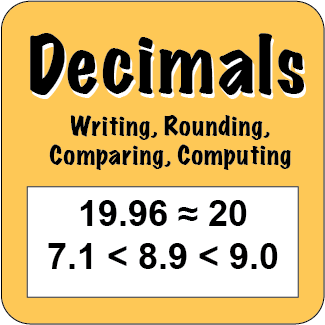Back to Mr. Anker TestsUse these practice activities to help you learn how to work with decimal values
in a number of ways, including: writing, rounding, comparing and computing.
Teachers & Parents: It is recommended that students complete and master each activity before moving on to the next.

 Decimals on a Number Line 1Represent on a number line fractions & decimals... Decimals on a Number Line 2Represent on a number line fractions & decimals... Fractions & Decimals 1Understand that fractions & decimals are two different ways to represent the same value. Fractions & Decimals 2Understand that fractions & decimals are two different ways to represent the same value.
 Rounding Decimals 1Round values under 10 to the nearest whole number. Rounding Decimals 2Round values under 100 to the nearest whole number. Rounding Decimals 3Round values under 100 to the nearest 10th. Rounding Decimals 4Round values under 100 to the nearest 100th.
 Rounding Decimals 5Round values with many decimal places to the nearest whole number. Rounding Decimals 6Round values with many decimal places to the nearest whole number, 10th and 100th. Rounding Decimals 7Round values with many decimal places to the nearest whole number, 10th and 100th. Rounding Decimals 8Round values with many decimal places to the nearest whole number, 10th and 100th.
 Writing Decimals 10thsWrite decimal values with words and numbers to the tenths place. Writing Decimals 100thsWrite decimal values with words and numbers to the hundredths place. Writing Decimals 1000thsWrite decimal values with words and numbers to the thousandth place. Writing Decimals MixedWrite decimal values with words and numbers to the tenths, hundredths, and thousandths place.